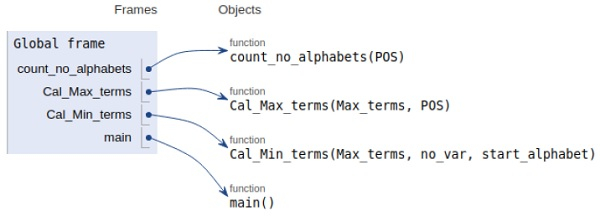# Python-program-to-convert-pos-to-sop

Problem statement − We are given pos form we need to convert it into its equivalent sop form

The conversion can be done by first counting the number of alphabets in the pos form and then calculating all the max and the minterms.

Now let’s observe the concept in the implementation below−

## Example

# Python code to convert standard POS form
# to standard SOP form
# to calculate number of variables
def count_no_alphabets(POS):
i = 0
no_var = 0
# total no. of alphabets before will be equal to alphabets before first '.' character
while (POS[i]!='.'):
# character is a alphabet or not
if (POS[i].isalpha()):
no_var+= 1
i+= 1
return no_var
# maximum terms in an integer
def Cal_Max_terms(Max_terms, POS):
a = ""
i = 0
while (i<len(POS)):
if (POS[i]=='.'):
# binary to decimal conversion
b = int(a, 2)
# append each min term(integer type) into the list
Max_terms.append(b)
# assign empty strings
a =""
i+= 1
elif(POS[i].isalpha()):
# checking whether variable is having complement as superscript
if(i + 1 != len(POS) and POS[i + 1]=="'"):
# concatenating the string with '1'
a += '1'
# incrementing by 2 because 1 for alphabet and another for a symbol "'"
i += 2
else:
# concatenating the string with '0'
a += '0'
i += 1
else:
i+= 1
# append last min term(integer type) into the list
Max_terms.append(int(a, 2))
# conversion of minterms in binary and finally converting it to SOP
def Cal_Min_terms(Max_terms, no_var, start_alphabet):
# declaration of the list
Min_terms =[]
# calculation of total no. of terms formed by all variables max = 2**no_var
for i in range(0, max):
# is current term present in max_terms or not
if (Max_terms.count(i)== 0):
# converting integer to binary
b = bin(i)[2:]
# loop used for inserting 0's before the
# binary value so that its length will be
# equal to no. of variables present in
# each product term
while(len(b)!= no_var):
b ='0'+b
# appending the max terms(integer) in the list
Min_terms.append(b)
SOP = ""
# iterated untill minterms are available
for i in Min_terms:
# fetching the variable
value = start_alphabet
# iterate till there are 0's and 1's
for j in i:
# check whether the varailble is complemented or not
if (j =='0'):
# concatenating vaue and complement operator
SOP = SOP + value+ "'"
# check the non complement variable
else:
# concatenating value
SOP = SOP + value
# increment the alphabet by the next adjacent alaphabet
value = chr(ord(value)+1)
# concatenating the "+" operator
SOP = SOP+ "+"
# for discarding the extra '+'
SOP = SOP[:-1]
return SOP
# main function
def main():
# input
POS_expr ="(A'+B'+C).(A+B+C').(A+B'+C).(A'+B+C)"
Max_terms = []
no_var = count_no_alphabets(POS_expr)
Cal_Max_terms(Max_terms, POS_expr)
SOP_expr = Cal_Min_terms(Max_terms, no_var, POS_expr)
print("Standard SOP form of " + POS_expr + " ==> " + SOP_expr)
# Driver code
if __name__=="__main__":
main()

## Output

Standard SOP form of (A'+B'+C).(A+B+C').(A+B'+C).(A'+B+C) ==>
A'B'C'+A'BC+AB'C+ABCAll the variables are declared in the local scope and their references are seen in the figure above.